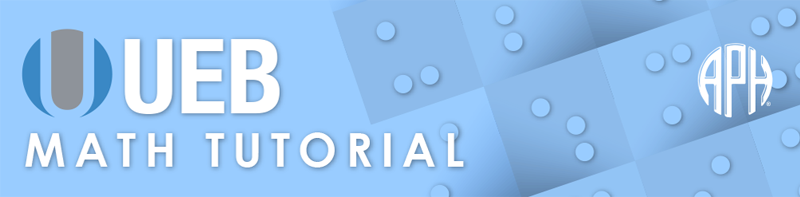Practice Problems -   -  Use 6 Dot Entry   Switch to Nemeth Tutorial

# Lesson 10.2: Integrals

## Symbols

$\int \phantom{\rule{.3em}{0ex}}\text{integral}$

$\oint \phantom{\rule{.3em}{0ex}}\text{closed line integral}$
⠈⠮

$\text{superposition}$

## Review

The scope of a level change indicator is the next item. Braille grouping indicators must be used to enclose an expression that includes more than one item.

When complex algebraic equations contain indicators such as superscript, subscript, fractions, radicals, and/or letters standing alone, it is best to enclose them in grade 1 passage indicators to ensure the symbols are well defined without the need for grade 1 symbol indicators. When a complex expression is comprised of a single symbol sequence, a grade 1 word indicator will be enough to ensure that the various indicators are well defined without the need for grade 1 symbol indicators.

## Explanation

An integral is a mathematical object that is used to calculate an area under a functions curve. In print the symbol used for integral notation is an elongated letter s. The integral limits may be displayed directly under and directly over, or in the subscript and superscript positions to the integral symbol. In braille it is recommended to treat the limits as subscripts and superscripts.

The integral symbol is formed with dots two three four six. The subscript is brailled first, followed by the superscript, and finally the element(s) to the right of the integral. The extent of the level change indicator is the next item. Braille grouping indicators are required if the expression extends beyond one item. The element directly to the right of the integral is brailled as it is shown in print, unspaced from the integral. The integral symbol requires grade 1 mode as do the subscript and superscript indicators. Grade 1 word mode is used unless the integral expression is part of an equation with three or more symbol sequences that require grade 1 passage mode. Grade 1 mode may also be turned on by the numeric indicator.

The integral sign may have shapes or symbols superimposed on it in print. A circle, square, rectangle or sign for infinity may be used. In braille, the symbol for a closed line integral (integral with a circle superimposed) is two cells; dot four, dots two three four six. Another shape or symbol superimposed on the integral will require the superposition indicator in braille, dots one two three four six followed by the signs for the shape or other symbol. The superposition indicator requires grade 1 mode. A shape terminator is needed with the shape indicator if there is no space after the shape.

### Example 1

$\int {x}^{2}\mathrm{dx}$
⠰⠰⠮⠭⠔⠼⠃⠰⠙⠭

### Example 2

${\int }_{1}^{2}{x}^{2}=\frac{7}{3}$
⠰⠰⠮⠢⠼⠁⠔⠼⠃⠭⠔⠼⠃⠀⠐⠶⠀⠼⠛⠌⠉

### Example 3

${\int }_{a}^{b}f\left(x\right)\mathrm{dx}$
⠰⠰⠮⠢⠁⠔⠃⠋⠐⠣⠭⠐⠜⠙⠭

### Example 4

$\int 3{x}^{2}\mathrm{dx}$
⠰⠮⠼⠉⠭⠔⠼⠃⠰⠙⠭

### Example 5

$\int \frac{1}{x}\mathrm{dx}$
⠰⠰⠮⠷⠼⠁⠨⠌⠭⠾⠙⠭

### Example 6

$5{\int }_{a}^{b}f\left(x\right)\mathrm{dx}$
⠼⠑⠮⠢⠁⠔⠃⠋⠐⠣⠭⠐⠜⠙⠭

### Example 7

${\int }_{0}^{\infty }x{e}^{-{x}^{2}}\mathrm{dx}$
⠰⠰⠮⠢⠼⠚⠔⠼⠿⠭⠑⠔⠣⠐⠤⠭⠔⠼⠃⠜⠙⠭

### Example 8

${\int }_{1}^{n}f\left(x\right)\mathrm{dx}<{\int }_{1}^{\infty }f\left(x\right)\mathrm{dx}$
⠰⠰⠰⠮⠢⠼⠁⠔⠝⠋⠐⠣⠭⠐⠜⠙⠭⠀⠈⠣⠀⠮⠢⠼⠁⠔⠼⠿⠋⠐⠣⠭⠐⠜⠙⠭⠰⠄

### Example 9

${\int }_{0}^{1}{\int }_{{x}^{2}}^{x}\left(x+3\right)\mathrm{dy}\mathrm{dx}$
⠰⠰⠮⠢⠼⠚⠔⠼⠁⠮⠢⠣⠭⠔⠼⠃⠜⠔⠭⠐⠣⠭⠐⠖⠼⠉⠐⠜⠙⠽⠙⠭

### Example 10

${\oint }_{C}M\mathrm{dx}+N\mathrm{dy}$
⠰⠰⠈⠮⠢⠠⠉⠠⠍⠙⠭⠐⠖⠠⠝⠙⠽